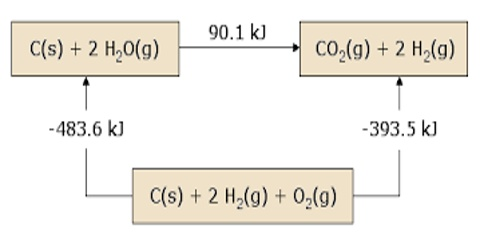# Hess’s Law of Thermochemistry

Hess’s Law of Thermochemistry

Two laws of thermochemistry are very important in thermochemical calculations. Both the laws preceded the first law of thermodynamics (1850). It will, however be shown that the laws of Thermochemistry are direct consequence of the first law of thermodynamics.

Hess’s law (1840)

This law was enunciated by G. H. Hess in 1840 and is also known as the law of constant heat summation. The law may be stated in different forms. The following statement of the law is used most often.

If a chemical reaction or physical change can be brought about in more than one ways, the overall enthalpy change is the same provided the initial and final states are the same.

This law is a manifestation that enthalpy is a state function. This means that if a reaction, in theory, can be carried out in a number of steps the enthalpy change for the overall reaction is the sum of the enthalpy changes for all the steps. Since H is a thermodynamic state function, ∆H for a reaction is merely the difference between the value of H in the final state (i.e. products) and the initial state (i.e., reactants).

The law can be illustrated by the following example:

When C (graphite) is burned in oxygen CO2 can be produced in one step. The same reaction can be carried out in two steps: in the first step C (graphite) is converted to CO (g) by reaction with O2 (g) and in the second step CO (g) is burned to CO2 (g) by reaction with more O2 (g). The reactions are shown below:

One step reaction: C (graphite) + O2 (g) → CO2 (g); heat charge = ∆H1

Two steps:       (i) step 1: C (graphite) + ½ O2 (g) → CO (g); heat change = ∆H2

(ii) step 2: CO (g) + ½ O2 (g) → CO2 (g); heat Change = ∆H3

According to Hess’s law: ∆H1 = ∆H2 + ∆H3

since in the two routes the final state and the initial state are the same as can be seen by adding the components in steps (i) and (ii). This can be shown mathematically as follows: